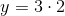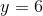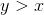# ISEE Middle Level Quantitative : How to find the points on a coordinate plane

## Example Questions

### Example Question #6 : Coordinate Geometry

Choose the best answer from the four choices given.

The point (15, 6) is on which of the following lines?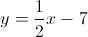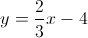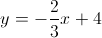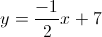Explanation:

For this problem, simply plug in the values for the point (15,6) into the different equations (15 for the-value and 6 for the-value) to see which one fits.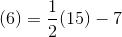(NO)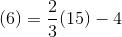(YES!)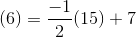(NO)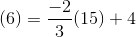(NO)

### Example Question #7 : Coordinate Geometry

Choose the best answer from the four choices given.

What is the point of intersection for the following two lines?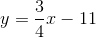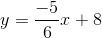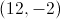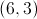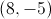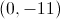Explanation:

At the intersection point of the two lines the- and- values for each equation will be the same. Thus, we can set the two equations as equal to each other: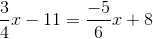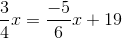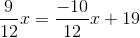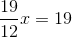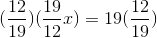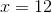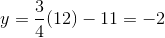point of intersection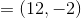### Example Question #8 : Coordinate Geometry

Choose the best answer from the four choices given.

What is the-intercept of the line represented by the equation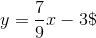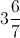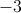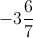Explanation:

In the formula, the y-intercept is represented by(because if you setto zero, you are left with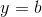).

Thus, to find the-intercept, set thevalue to zero and solve for.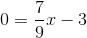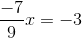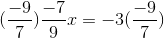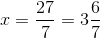### Example Question #9 : Coordinate Geometry

The ordered pair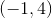is in which quadrant?

Explanation:

There are four quadrants in the coordinate plane. Quadrant I is the top right, and they are numbered counter-clockwise. Since the x-coordinate is, you go to the left one unit (starting from the origin). Since the y-coordinate is, you go upwards four units. Therefore, you are in Quadrant II.

### Example Question #10 : Coordinate Geometry

If angles s and r add up to 180 degrees, which of the following best describes them?

Complementary

Obtuse

Acute

Supplementary.

Supplementary.

Explanation:

Two angles that are supplementary add up to 180 degrees. They cannot both be acute, nor can they both be obtuse. Therefore, "Supplementary" is the correct answer.

### Example Question #1 : How To Find The Points On A Coordinate Plane

The lines of the equations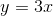and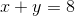intersect at a point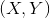.

Which is the greater quantity?

(a)(b)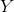(a) is the greater quantity

It is impossible to determine which is greater from the information given

(a) and (b) are equal

(b) is the greater quantity

(b) is the greater quantity

Explanation:

Ifand, we can substitute in the second equation as follows: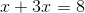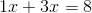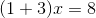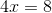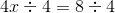Substitute: